Author: Oscar Cronquist Article last updated on September 27, 2022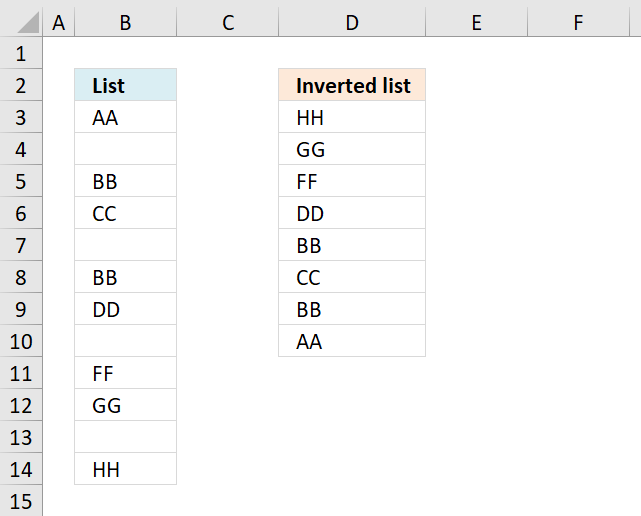This article demonstrates two different formulas, one for Excel 365 and one for earlier versions.

## 1. Reverse a list ignoring blanks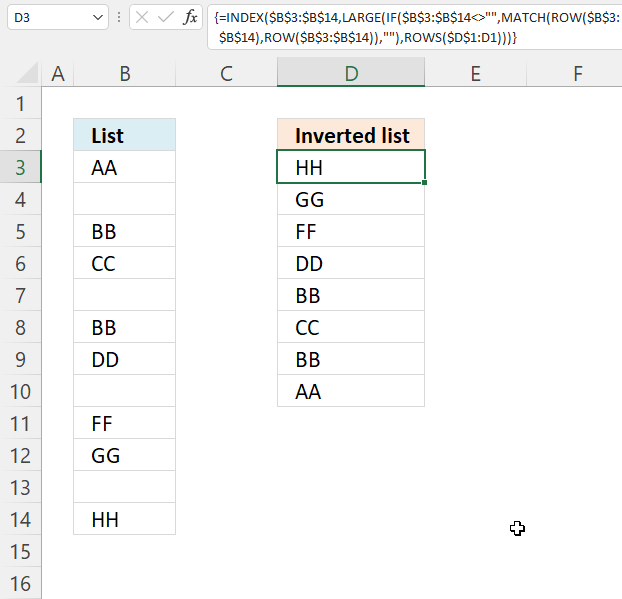The image above demonstrates a formula in cell D3 that rearranges values, bottom value is now on top etc.

Formula in C2:

=INDEX(\$B\$3:\$B\$14, LARGE(IF(\$B\$3:\$B\$14<>"", MATCH(ROW(\$B\$3:\$B\$14), ROW(\$B\$3:\$B\$14)), ""), ROWS(\$D\$1:D1)))

To enter an array formula, type the formula in a cell then press and hold CTRL + SHIFT simultaneously, now press Enter once. Release all keys.

The formula bar now shows the formula with a beginning and ending curly bracket telling you that you entered the formula successfully. Don't enter the curly brackets yourself.

### Explaining formula in cell D3

#### Step 1 - Find non-empty cells

\$B\$3:\$B\$14<>""

becomes

{"AA"; ""; "BB"; "CC"; ""; "BB"; "DD"; ""; "FF"; "GG"; ""; "HH"}<>""

and returns

{TRUE; FALSE; TRUE; TRUE; FALSE; TRUE; TRUE; FALSE; TRUE; TRUE; FALSE; TRUE}

#### Step 2 - Replace TRUE with corresponding row value

The IF function returns the row number if cell is not blank. FALSE returns "" (nothing).

IF(\$B\$3:\$B\$14<>"", MATCH(ROW(\$B\$3:\$B\$14), ROW(\$B\$3:\$B\$14)), "")

becomes

IF({TRUE; FALSE; TRUE; TRUE; FALSE; TRUE; TRUE; FALSE; TRUE; TRUE; FALSE; TRUE}, MATCH(ROW(\$B\$3:\$B\$14), ROW(\$B\$3:\$B\$14)), "")

becomes

IF({TRUE; FALSE; TRUE; TRUE; FALSE; TRUE; TRUE; FALSE; TRUE; TRUE; FALSE; TRUE}, {1;2;3;4;5;6;7;8;9;10;11;12}, "")

and returns

{1;"";3;4;"";6;7;"";9;10;"";12}

#### Step 3 - Find k-th largest row number

The LARGE function extracts the k-th smallest number from cell range or array. LARGE(array. k) The second argument contains ROWS(\$D\$1:D1), it has an expanding cell reference that grows when cell is copied to cells below.

LARGE(IF(\$B\$3:\$B\$14<>"", MATCH(ROW(\$B\$3:\$B\$14), ROW(\$B\$3:\$B\$14)), ""), ROWS(\$D\$1:D1))

becomes

LARGE({1;"";3;4;"";6;7;"";9;10;"";12}, ROWS(\$D\$1:D1))

becomes

LARGE({1;"";3;4;"";6;7;"";9;10;"";12}, 1)

and returns 12.

#### Step 4 - Return value

The INDEX function returns a value based on a row and column number. In this formula the cell range is only in one column, the row number is only needed.

INDEX(\$B\$3:\$B\$14, LARGE(IF(\$B\$3:\$B\$14<>"", MATCH(ROW(\$B\$3:\$B\$14), ROW(\$B\$3:\$B\$14)), ""), ROWS(\$D\$1:D1)))

becomes

INDEX(\$B\$3:\$B\$14, 12)

and returns "HH" in cell D3.

### Get Excel *.xlsx file

Invert a list ignoring blanks.xlsx

## 2. Reverse a list ignoring blanks - Excel 365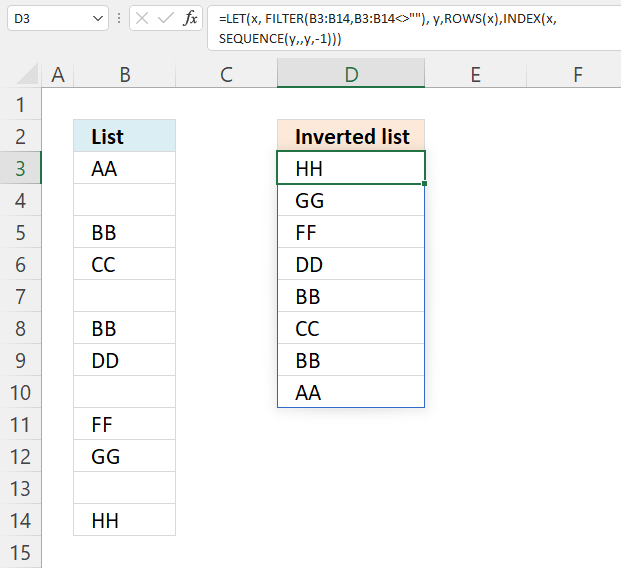This example shows an Excel 365 formula that extracts values in cell range B3:B14, ignores blank cells and returns the values starting from the bottom.

Excel 365 dynamic array formula in cell D3:

=LET(x, FILTER(B3:B14, B3:B14<>""), y, ROWS(x), INDEX(x, SEQUENCE(y, , y, -1)))

### 2.1 Explaining formula

#### Step 1 - Logical expression

The less than and larger than characters combined lets you filter non-empty values, the result is a boolean value TRUE or FALSE.

B3:B14<>""

becomes

{"AA"; ""; "BB"; "CC"; ""; "BB"; "DD"; ""; "FF"; "GG"; ""; "HH"}<>""

and returns

{TRUE; FALSE; TRUE; TRUE; FALSE; TRUE; TRUE; FALSE; TRUE; TRUE; FALSE; TRUE}.

#### Step 2 - Filter list excluding blanks

The FILTER function lets you extract values/rows based on a condition or criteria.

FILTER(arrayinclude, [if_empty])

FILTER(B3:B14, B3:B14<>"")

becomes

FILTER({"AA"; ""; "BB"; "CC"; ""; "BB"; "DD"; ""; "FF"; "GG"; ""; "HH"}, {TRUE; FALSE; TRUE; TRUE; FALSE; TRUE; TRUE; FALSE; TRUE; TRUE; FALSE; TRUE})

and returns

{"AA"; "BB"; "CC"; "BB"; "DD"; "FF"; "GG"; "HH"}.

#### Step 3 - Calculate the number of values in the array

The ROWS function returns the number of rows a given reference or array contains.

ROWS(ref)

ROWS(FILTER(B3:B14,B3:B14<>""))

becomes

ROWS({"AA"; "BB"; "CC"; "BB"; "DD"; "FF"; "GG"; "HH"})

and returns 8.

#### Step 4 - Create a sequential list of numbers from n to 1

The SEQUENCE function creates a list of sequential numbers.

SEQUENCE(rows, [columns], [start], [step])

SEQUENCE(ROWS(FILTER(B3:B14, B3:B14<>"")), , ROWS(FILTER(B3:B14, B3:B14<>"")), -1)

becomes

SEQUENCE(8, , 8, -1)

and returns {8; 7; 6; 5; 4; 3; 2; 1}.

#### Step 5 - Get values backwards

The INDEX function returns a value from a cell range, you specify which value based on a row and column number.

INDEX(array[row_num][column_num], [area_num])

INDEX(FILTER(B3:B14,B3:B14<>""),SEQUENCE(ROWS(FILTER(B3:B14,B3:B14<>"")),,ROWS(FILTER(B3:B14,B3:B14<>"")),-1))

becomes

INDEX({"AA"; "BB"; "CC"; "BB"; "DD"; "FF"; "GG"; "HH"}, {8; 7; 6; 5; 4; 3; 2; 1})

and returns

{"HH"; "GG"; "FF"; "DD"; "BB"; "CC"; "BB"; "AA"}.

#### Step 6 - Optimize formula

The LET function lets you name intermediate calculation results which can shorten formulas considerably and improve performance.

LET(name1name_value1calculation_or_name2, [name_value2calculation_or_name3...])

INDEX(FILTER(B3:B14,B3:B14<>""),SEQUENCE(ROWS(FILTER(B3:B14,B3:B14<>"")),,ROWS(FILTER(B3:B14,B3:B14<>"")),-1))

FILTER(B3:B14, B3:B14<>"") is repeated three times in the formula.

x - FILTER(B3:B14, B3:B14<>"")

ROWS(FILTER(B3:B14, B3:B14<>"")) is repeated two times in the formula.

y - ROWS(x)

LET(x, FILTER(B3:B14, B3:B14<>""), y, ROWS(x), INDEX(x, SEQUENCE(y, , y, -1)))

## 3. Reverse a list ignoring blanks - manual steps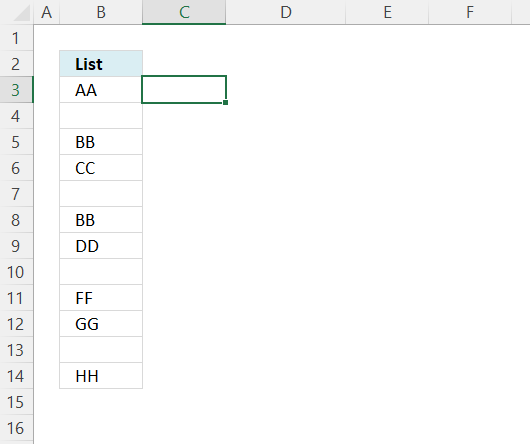Create a list of numbers next to the list or table.

1. Type 1 in the first cell.
2. Type 2 in the next cell below.
3. Select both cells.
4. Press and hold with left mouse button on the dot in the lower right corner of the selection.
5. Drag with mouse as far as needed.
6. Release left mouse button.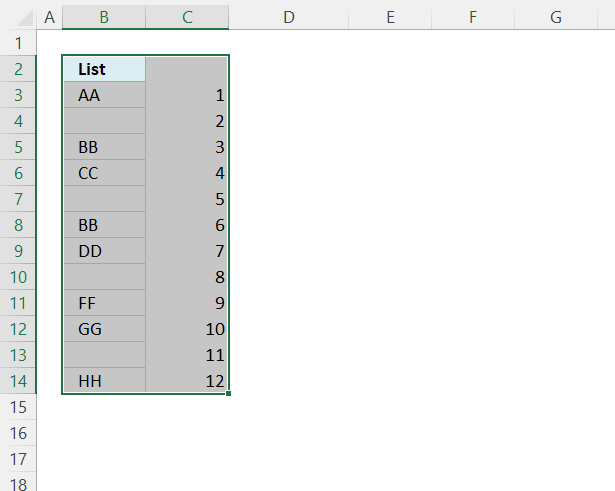1. Select all cells, see the image above.
2. Press CTRL + SHIFT + L to apply the Excel FILTER feature. Filter buttons appear at the top cells.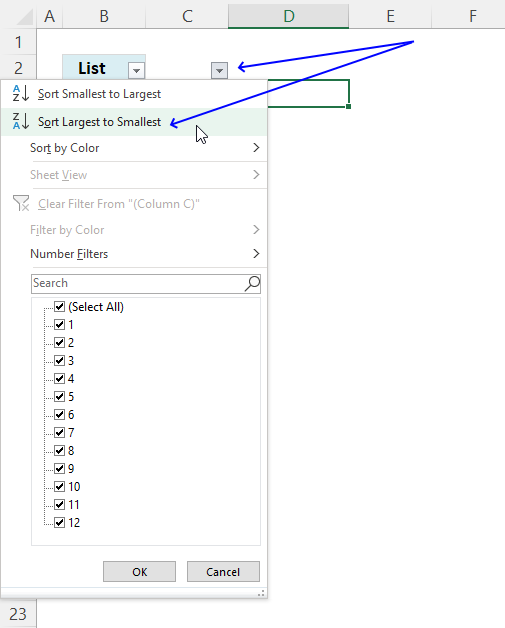3. Press with left mouse button on the right button, a popup menu appears.
4. Press with mouse on "Sort Largest to Smallest".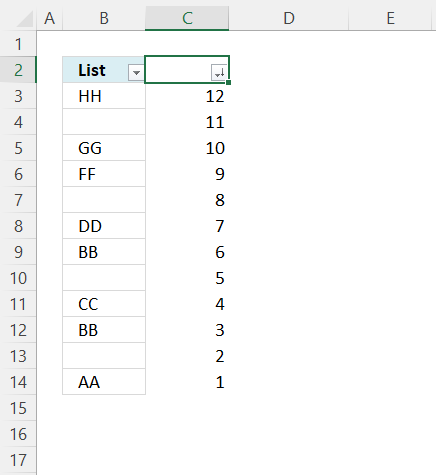1. Press with left mouse button on the left button, and a popup menu appears.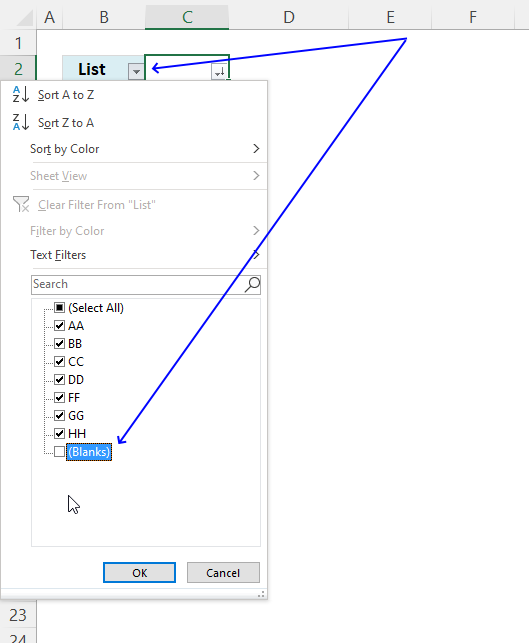2. Press with left mouse button on the check box next to blanks to deselect it, see the image above.
3. Press with left mouse button on the OK button.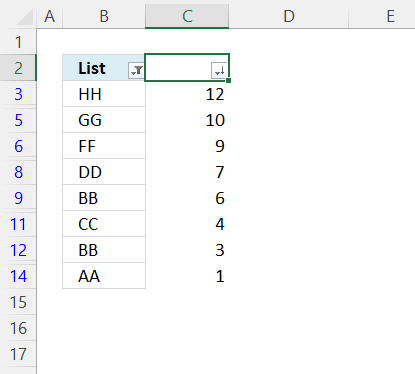Press CTRL + SHIFT + L to disable the Excel Filter. Delete the numbers next to the list.